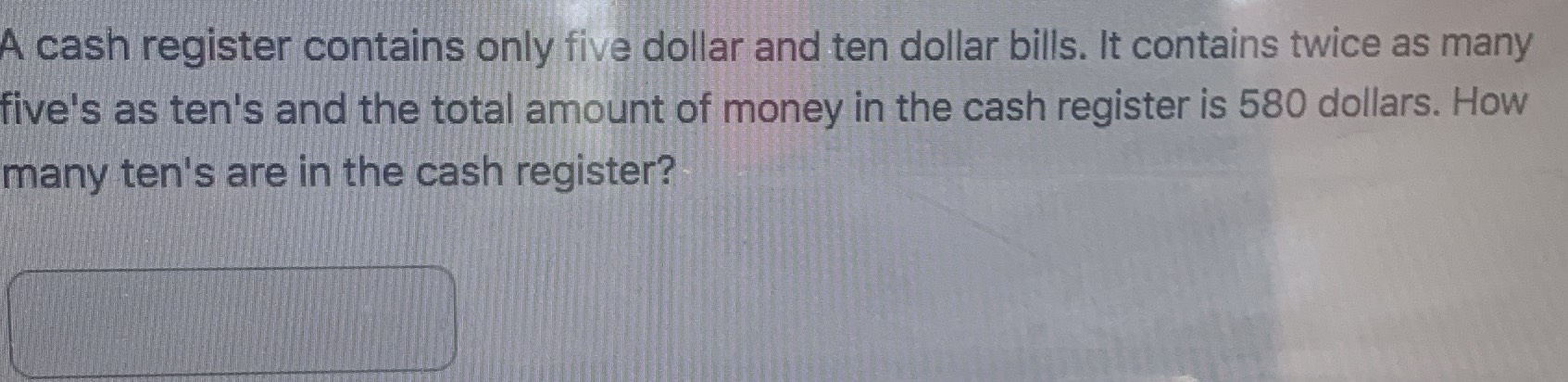### Still have math questions?

Algebra
QuestionA cash register contains only five dollar and ten dollar bills. It contains twice as many five's as ten's and the total amount of money in the cash register is $$580$$ dollars. How many ten's are in the cash register?

Let's call 5 dollar bills x and ten dollar bills y.

x = 2y

Now for the money part. The fives will be 5x (five dollars times the number of 5 dollar bills). The tens will be 10y.

5x + 10y = 580.

But you can't solve an equation with two variables. So somehow we need to get rid of either x or y in one of the equations. Well, according to our first equation, x is the same thing as 2y. So in the second equation, we can use 2y instead of x.

5(2y) + 10y = 580

Now I can solve for y.

10y + 10y = 580

Combine like terms. 20y = 580

Divide both sides by 20.

y = 29

So there are 29 ten dollar bills.

Solution
View full explanation on CameraMath App.